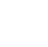Back to Chapter
C++ Setting UpC++ IntroductionNo items found.
C++ BasicsNo items found.
C++ Control StatementsNo items found.
C++ Logical OperatorsNo items found.
C++ Procedural ProgrammingNo items found.
C++ Structural ProgrammingNo items found.
C++ Implementation of OOPSNo items found.
C++ Arrays and VectorsNo items found.
C++ Error HandlingNo items found.
C++ File HandlingNo items found.

# C++ Inline Functions and Recursion

### Inline Functions

C++ inline function is very useful concept that is commonly used with classes. If a function is inline, the compiler places a copy of the code of that function at each point where the function is called at compile time.

Basically an inline function code is re-written wherever the function call is encountered.

``` #include using namespace std; inline int greater(int x, int y) { return (x > y)? x : y; } // Main function for the program int main() { cout << "Max (20,10): " << greater(20,10) << endl; cout << "Max (0,200): " << greater(0,200) << endl; cout << "Max (100,1010): " << greater(100,1010) << endl; return 0; } ```
``` Output: Max (20,10): 20 Max (0,200): 200 Max (100,1010): 1010 ```

### Recursion

When function is called within the same function, it is known as recursion in C++. Using recursive algorithm, certain problems can be solved quite easily.

Consider a situation where we have to determine the factorial of first n natural numbers. Lets try to write a program for the same.

Factorial of number is basically the product of the number and product of all numbers preceding the number. So factorial of 6 will be 1*2*3*4*5*6=720

To perform this we can use a do while loop.

``` #inclide using namespace std; main() { int n; int factorial=1; cout<<"enter the number:"; cin>>n; do { factorial=factorial*n;//multiplying n and n-1 n--;//decrementing n by 1 }while(n!=0); cout<<"\nfactorial:"<```
``` Output: enter the number:6 factorial:720 ```

`We can perform the same operation using a recursive function.`

`Looking at the above flow chart we can write a recursive function using the given syntax`

``` void exampleRecursive() { ....... condition true{ exampleRecursive; } return ; } ```

`Now lets write a code for factorial of a number using recursive function.`

``` #include using namespace std; int factorialRecursive(int n) { if(n > 1) return n * factorialRecursive(n - 1); else return 1; } int main() { int n; cout << "Enter a positive integer: "; cin >> n; cout << "Factorial of " << n << " = " << factorial(n); return 0; } ```
``` Output: Enter a positive integer: 5 Factorial of 5 = 120 ```

`‍`

`‍`

`‍`

`Caution! Recursion is very useful in certain situations but the use of recursion must be avoided unless you are very confident about what you are doing, recursion can wield undesired results if the code is not specific.`
`Menu`
`HomeCoursesEventsCampus Ambassador Program`
`About`
`About UsPrivacy PolicyTerms and ConditionsCancellation and Refund PolicyContact UsCareers`
`Courses`
`Full-Stack Web Dev Industrial Training Program`
`Data Scientist Industrial Training Program`
`DevTown Bytes`
`RoadmapsCheatsheetsVideosBlogs`
``` ```
``````
```Ask queriesContact Us on WhatsappHi, How Can We Help You? ```